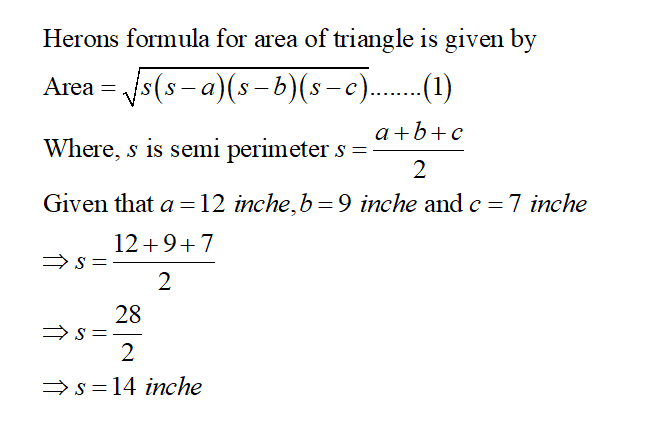# Using Heron's formula, calculate the area of a triangle with side lengths of 12, 9, and 7 inches. Round to the nearest tenth.

Question
1 views

Using Heron's formula, calculate the area of a triangle with side lengths of 12, 9, and 7 inches. Round to the nearest tenth.

check_circle

Step 1...

### Want to see the full answer?

See Solution

#### Want to see this answer and more?

Solutions are written by subject experts who are available 24/7. Questions are typically answered within 1 hour.*

See Solution
*Response times may vary by subject and question.
Tagged in